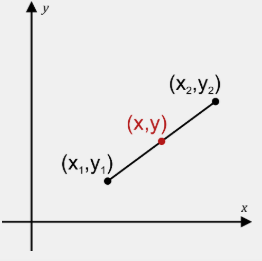# Midpoint Calculator

Using our Midpoint Calculator can be the easiest and most convenient way to calculate the midpoint. You just need to give the input coordinates in the input field and click on the enter button to get the concerned output.

Midpoint Calculator

Midpoint Calculator: Finding Midpoint in geometry is often required. In such cases, Handy Tool over here will give you the midpoint instantaneously if you give the input coordinates. Apart from plotting the midpoint of the segment, this online calculator will generate a step by step explanation on how the result arrived. Continue reading to know what is meant by Midpoint and how to calculate the midpoint. This free online tool will display the midpoint of the line segment in the blink of an eye.

Contents

## What is meant by Midpoint?

In Maths, the midpoint is defined as the middle point of the line segment. It should be equidistant from both the endpoints of the line segment. In fact, it is halfway for the line segment and in other words, it can be referred to as the point that divides a line segment into two equal segments. Any line segment will have a unique midpoint.

### How to find Midpoint?

Suppose we have a line segment and want to cut it to two equal parts then we need to know the center. We can do this by simply knowing the midpoint. Midpoint is simply the average of each coordinate of the section forming a new coordinate point.

• Label the coordinates (X1, Y1) and (X2, Y2)
• Add the values in the Parentheses and divide each result by 2.
• New values form the new coordinates of the midpoint.Formula to obtain the midpoint is given as

 (X, Y) = [(X1 + X2)/2, (Y1 + Y2)/2]

where X1, X2 are the coordinates of X-Axis and Y1, Y2 are the coordinates of Y-Axis.

Quickly learn different concepts of maths with the help of free tools available at onlinecalculator.guru that give you a detailed explanation on each mathematical concept.

### FAQs on Midpoint

1.  What is Midpoint used for?

Midpoint is used to find the exact center point between two defined points.

2. How do you find the Midpoint easily?

You can find the midpoint easily even for larger numbers too by taking the help of Midpoint Calculator.

3. What is the formula to find Midpoint between two points?

Formula to find Midpoint between two points is (X, Y) = [(X1 + X2)/2, (Y1 + Y2)/2]

4. Where do I find detailed steps on how to find midpoint?

You can find detailed steps on how to find midpoint on our page.

### Finding Midpoint for the Coordinates (4,12) and (3,5)

Elaborate Solution to find the midpoint of coordinates (4,12) and (3,5)

Utilize the Midpoint Calculator to evaluate the midpoint of coordinates (4,12) and (3,5) i.e. (3.5, 8.5) along with a detailed work on how the result arrived.

1. What is the midpoint of coordinates (4,12) and (3,5)?

The midpoint of Coordinates (4,12) and (3,5) is (3.5, 8.5).

2. How to find the Midpoint of Coordinates (4,12) and (3,5)?

Substitute the coordinates in the formula to find midpoint i.e. (X, Y) = [(X1 + X2)/2, (Y1 + Y2)/2]

(X, Y) = [(4+3)/2,(12+5)/2]

= [(7/2, 17/2)]

= (3.5,8.5)

Therefore, Midpoint of (4,12) and (3,5) is (3.5,8.5)

3. Where do I get detailed steps to find midpoint of (4,12) and (3,5) ?

You can get detailed steps on how to find the midpoint of (4,12) and (3,5)  on our page.